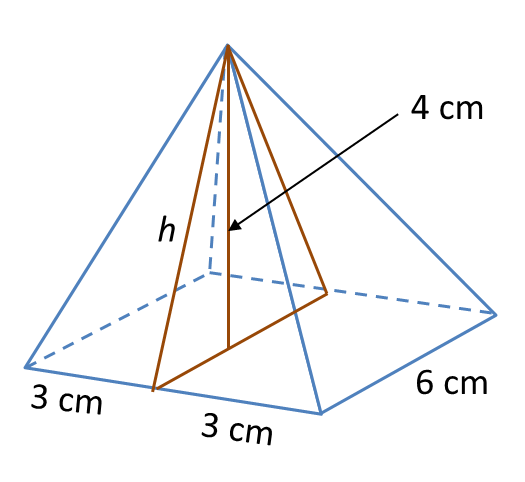# 6.4.2 Surface Area of Three Dimensional Shapes, PT3 Focus Practice

  
                            
6.4.2 Surface Area of Three Dimensional Shapes, PT3 Focus Practice
  
   Question 4:
   Sphere below has a surface area of 221.76 cm2.Calculate its radius.
                                $\left(\pi =\frac{22}{7}\right)$                             
  
   Solution:
   Surface area of sphere = 4πr2
                                $\begin{array}{l}4\pi {r}^{2}=221.76\\ 4×\frac{22}{7}×{r}^{2}=221.76\\ {r}^{2}=\frac{221.76×7}{4×22}\\ {r}^{2}=17.64\\ r=\sqrt{17.64}\\ r=4.2\text{cm}\end{array}$                           
  
  
  
  
                            
Question 5:
   Diagram below shows a right pyramid with a square base.Given the height of the pyramid is 4 cm.
   Calculate the total surface area, in cm2, of the right pyramid.
  (adsbygoogle = window.adsbygoogle || []).push({}); 
   Solution:h2 = 32 + 42
   = 9 + 16
   = 25
   h = √25   = 5 cm2
  
   Total surface area of the right pyramid
   = Base area + 4 (Area of triangle)
   = (6 × 6) + 4 × 4 (½ × 6 × 5)
   = 36 + 60
   = 96 cm2